# Transistor Biasing: What is it? (Circuits And Types of Transistor Biasing)

Contents

## What is Transistor Biasing?

Transistor Biasing is the process of setting a transistor’s DC operating voltage or current conditions to the correct level so that any AC input signal can be amplified correctly by the transistor.

Transistors are one of the most widely used semiconductor devices which are used for a wide variety of applications, including amplification and switching. However, to achieve these functions satisfactorily, a transistor must be supplied with a certain amount of current and/or voltage.

The process of setting these conditions for a transistor circuit is referred to as transistor biasing. Transistor biasing can be accomplished by various techniques that give rise to different kinds of biasing circuits.

However, all of these circuits are based on the principle of providing the right amount of base current, IB, and, in turn, the collector current, IC from the supply voltage, VCC when no signal is present at an input.

Moreover, the collector resistor RC has to be chosen so that the collector-emitter voltage, VCE, remains greater than 0.5V for transistors made of germanium and greater than 1V for the transistors made of silicon. A few of the ample biasing circuits are explained below.

The types of transistor biasing include:

• Fixed Base Bias or Fixed Resistance Bias
• Collector Feedback Bias
• Dual Feedback Bias
• Fixed Bias with Emitter Resistor
• Emitter Bias
• Emitter Feedback Bias
• Voltage Divider Bias

## Types of Transistor Biasing

### Fixed Base Bias or Fixed Resistance Bias

The biasing circuit shown in Figure 1 has a base resistor RB connected between the base and the VCC. Here the base-emitter junction of the transistor is forward biased by the voltage drop across RB, which is the result of IB flowing through it. From the figure, the mathematical expression for IB is obtained as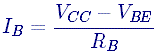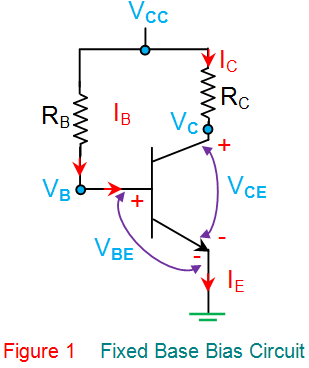Here the values of VCC and VBE are fixed, while the value for RB is constant once the circuit is designed.

This leads to a constant value for IB, resulting in a fixed operating point due to which the circuit is named fixed base bias. This kind of bias results in a stability factor of (β+1), leading to inferior thermal stability.

The reason behind this is the fact the β-parameter of a transistor is unpredictable and varies up to a large extent, even in the case of a transistor with the same model and type. This variation in β results in large changes in IC, which cannot be compensated by any means in the proposed design.

Hence, this kind of β dependent bias is prone to the changes in operating points brought about by the variations in transistor characteristics and temperature.

However, it is to be noted that fixed base bias is most simple and uses fewer components. Moreover, it offers the chance for the user to change the operating point anywhere in the active region by changing the value of RB in the design.

Furthermore, it offers no load on the source as there is no resistor across the base-emitter junction. Due to these factors, this kind of biasing is used in switching applications and to achieve automatic gain control in the transistors.

Here, the expressions for other voltages and currents are given as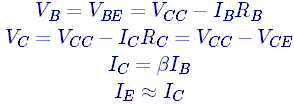### Collector Feedback Bias

In this circuit (Figure 2), the base resistor RB is connected across the collector and the base terminals of the transistor.

This means that the base voltage, VB, and the collector voltage, VC are inter-dependent because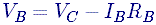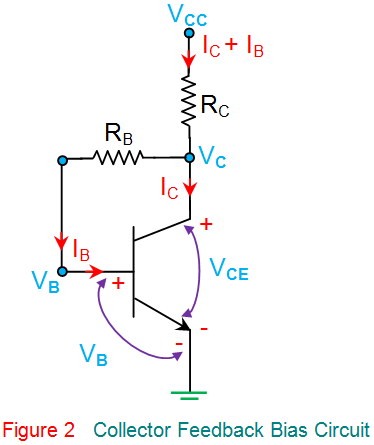Where,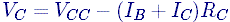From these equations, it is seen that an increase in IC decreases VC, which results in a reduced IB, automatically reducing IC.

This indicates that, for this type of biasing network, the Q-point (operating point) remains fixed irrespective of the variations in the load current causing the transistor to always be in its active region regardless of β value.

Furthermore, this circuit is also referred to as a self-biasing negative feedback circuit as the feedback is from output to input via RB.

This kind of relatively simple bias has a stability factor that is less than (β+1), which results in better stability when compared to fixed bias.

However, the action of reducing the collector current by base current leads to a reduced amplifier gain.

Here, other voltages and currents are expressed as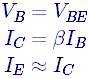### Dual Feedback Bias

Figure 3 shows a dual feedback bias network which is an improvisation over the collector feedback biasing circuit as it has an additional resistor R1 which increases the stability of the circuit.

This is because an increase in the current flow through the base resistors results in a network resistant to the variations in the values of β.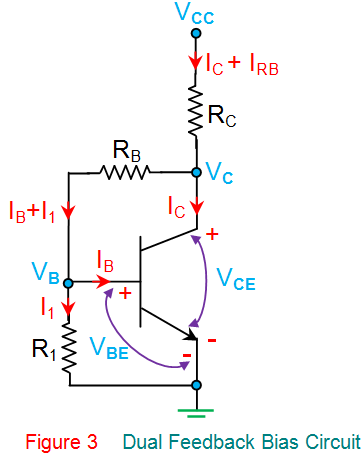Here,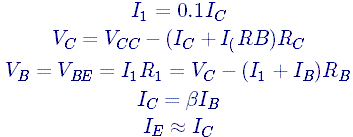### Fixed Bias with Emitter Resistor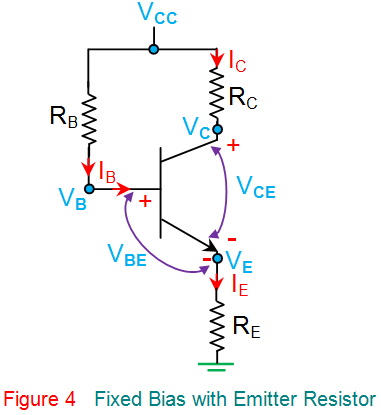As evident from Figure 4, this biasing circuit is nothing but a fixed bias network with an additional emitter resistor, RE.

Here, if IC rises due to an increase in temperature, the IE also increases, increasing the voltage drop across RE.

This results in the reduction of VC, causing a decrease in IB, bringing IC back to its normal value. Thus, this kind of biasing network offers better stability compared to a fixed base bias network.

However, the presence of RE reduces the voltage gain of the amplifier as it results in unwanted AC feedback. In this circuit, the mathematical equations for different voltages and current are given as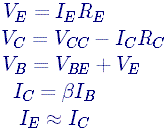### Emitter Bias

This biasing network (Figure 5) uses two supply voltages, VCC and VEE, equal but opposite in polarity.

Here VEE forward biases the base-emitter junction through RE while VCC reverse biases the collector-base junction. Moreover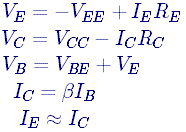In this kind of biasing, IC can be independent of both β and VBE by choosing RE >> RB/β and VEE >> VBE, respectively, which results in a stable operating point.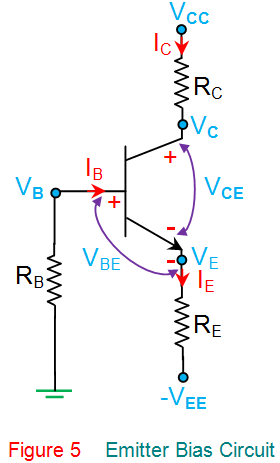### Emitter Feedback Bias

This kind of self-emitter bias (Figure 6) employs both collector-base feedback and emitter feedback to result in higher stability. Here, the emitter-base junction is forward biased by the voltage drop occurring across the emitter resistor, RE due to the flow of emitter current, IE.

An increase in the temperature increases IC, causing an increase in the emitter current, IE. This also leads to an increase in the voltage drop across RE which decreases the collector voltage, VC, and in turn IB, thereby bringing back IC to its original value.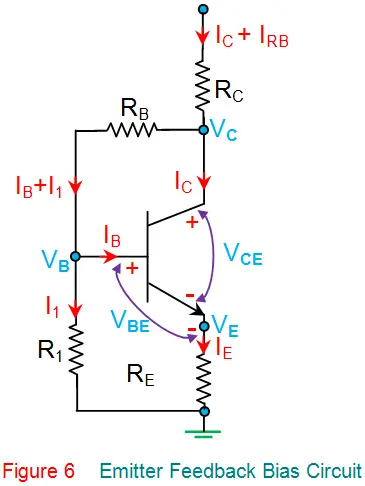However, this results in a reduced output gain due to degenerative feedback, which is nothing but unwanted AC feedback, wherein the amount of current flowing through the feedback resistor is determined by the value of the collector voltage, VC.

This effect can be compensated by using a large bypass capacitor across the emitter resistor, RE. The expressions corresponding to various voltages and currents in this low-power-supply-voltage suitable biasing network are given as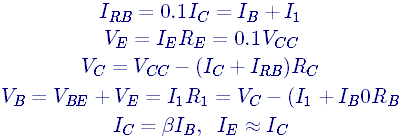### Voltage Divider Bias

This type of biasing network (Figure 7) employs a voltage divider formed by the resistors R1 and R2 to bias the transistor.

This means that the voltage developed across R2 will be the base voltage of the transistor, which forward biases its base-emitter junction. In general, the current through R2 will be fixed to be 10 times the required base current, IB (i.e., I2 = 10IB).

This is done to avoid its effect on the voltage divider current or the changes in β. Further, from the circuit, one gets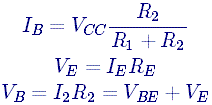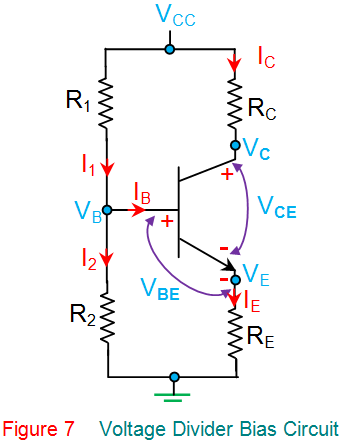In this kind of biasing, IC is resistant to the changes in both β as well as VBE, which results in a stability factor of 1 (theoretically), the maximum possible thermal stability.

As IC increases due to a temperature rise, IE increases, causing an increase in the emitter voltage VE, reducing the base-emitter voltage VBE. This results in the decrease of base current IB, which restores IC to its original value.

The higher stability offered by this biasing circuit makes it most widely used despite providing a decreased amplifier gain due to the presence of RE.

Apart from the analyzed basic types of biasing networks, Bipolar Junction Transistors (BJTs) can also be biased using active networks or by using either silicon or Zener diodes.

Furthermore, it is also to be noted that although the biasing circuits are explained for BJTs, similar bias networks also exist in the case of Field Effect Transistors (FETs).

Want To Learn Faster? 🎓
Get electrical articles delivered to your inbox every week.
No credit card required—it’s 100% free.